# FirstOrder Logic FOL aka predicate calculus FirstOrder Logic

• Slides: 13First-Order Logic (FOL) aka. predicate calculus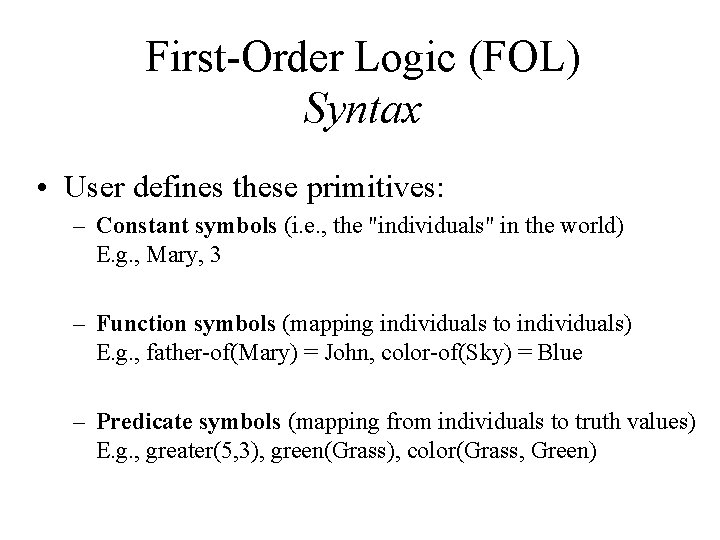First-Order Logic (FOL) Syntax • User defines these primitives: – Constant symbols (i. e. , the "individuals" in the world) E. g. , Mary, 3 – Function symbols (mapping individuals to individuals) E. g. , father-of(Mary) = John, color-of(Sky) = Blue – Predicate symbols (mapping from individuals to truth values) E. g. , greater(5, 3), green(Grass), color(Grass, Green)First-Order Logic (FOL) Syntax… • FOL supplies these primitives: – Variable symbols. E. g. , x, y – Connectives. Same as in PL: not (~), and (^), or (v), implies (=>), if and only if (<=>) – Quantifiers: Universal (A) and Existential (E)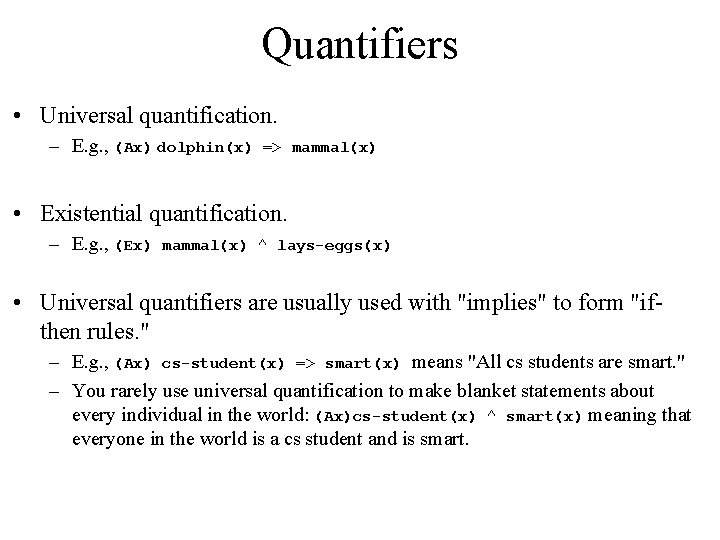Quantifiers • Universal quantification. – E. g. , (Ax) dolphin(x) => mammal(x) • Existential quantification. – E. g. , (Ex) mammal(x) ^ lays-eggs(x) • Universal quantifiers are usually used with "implies" to form "ifthen rules. " – E. g. , (Ax) cs-student(x) => smart(x) means "All cs students are smart. " – You rarely use universal quantification to make blanket statements about every individual in the world: (Ax)cs-student(x) ^ smart(x) meaning that everyone in the world is a cs student and is smart.Quantifiers … • Existential quantifiers are usually used with "and" to specify a list of properties or facts about an individual. – E. g. , (Ex) cs-student(x) ^ smart(x) means "there is a cs student who is smart. " – A common mistake is to represent this English sentence as the FOL sentence: (Ex) cs-student(x) => smart(x)Translating English to FOL • Every gardener likes the sun. (Ax) gardener(x) => likes(x, Sun) • You can fool some of the people all of the time. (Ex)(At) (person(x) ^ time(t)) => can-fool(x, t) • You can fool all of the people some of the time. (Ax)(Et) (person(x) ^ time(t) => can-fool(x, t) • All purple mushrooms are poisonous. (Ax) (mushroom(x) ^ purple(x)) => poisonous(x)Translating English to FOL… • No purple mushroom is poisonous. ~(Ex) purple(x) ^ mushroom(x) ^ poisonous(x) or, equivalently, (Ax) (mushroom(x) ^ purple(x)) => ~poisonous(x) • Deb is not tall. ~tall(Deb) • X is above Y if X is on directly on top of Y or else there is a pile of one or more other objects directly on top of one another starting with X and ending with Y. (Ax)(Ay) above(x, y) <=> (on(x, y) v (Ez) (on(x, z) ^ above(z, y)))Inference Rules for FOL • Inference rules for PL apply to FOL as well. For example, Modus Ponens, And-Introduction, And-Elimination, etc. • Generalized Modus Ponens (GMP) – Combines And-Introduction, Universal-Elimination, and Modus Ponens – E. g. : from P(c), Q(c), and (Ax)(P(x) ^ Q(x)) => R(x), derive R(c) – A substitution list Theta = {v 1/t 1, v 2/t 2, . . . , vn/tn} means to replace all occurrences of variable symbol vi by term ti. • Substitutions are made in left-to-right order in the list. • E. g. : subst({x/Ice. Cream, y/Ziggy}, eats(y, x)) = eats(Ziggy, Ice. Cream)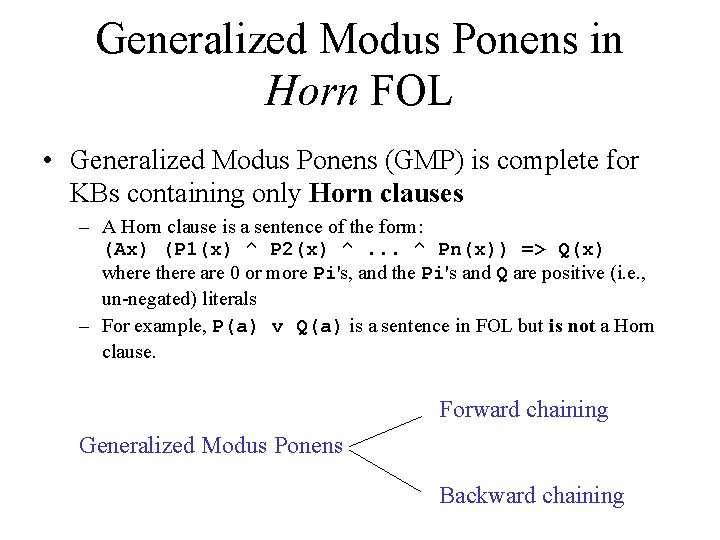Generalized Modus Ponens in Horn FOL • Generalized Modus Ponens (GMP) is complete for KBs containing only Horn clauses – A Horn clause is a sentence of the form: (Ax) (P 1(x) ^ P 2(x) ^. . . ^ Pn(x)) => Q(x) where there are 0 or more Pi's, and the Pi's and Q are positive (i. e. , un-negated) literals – For example, P(a) v Q(a) is a sentence in FOL but is not a Horn clause. Forward chaining Generalized Modus Ponens Backward chainingForward Chaining • Natural deduction using GMP is complete for KBs containing only Horn clauses. • Proofs start with the given axioms in KB, deriving new sentences using GMP until the goal/query sentence is derived. • This defines a forward chaining inference procedure because it moves "forward" from the KB to the goal.Example of forward chaining • Example: KB = All cats like fish, cats eat everything they like, and Ziggy is a cat. In FOL, KB = 1. 2. 3. • (Ax) cat(x) => likes(x, Fish) (Ax)(Ay) (cat(x) ^ likes(x, y)) => eats(x, y) cat(Ziggy) Goal query: Does Ziggy eat fish? Proof: Data-driven 1. Use GMP with (1) and (3) to derive: 4. likes(Ziggy, 2. Use GMP with (3), (4) and (2) to derive eats(Ziggy, 3. So, Yes, Ziggy eats fish. Fish)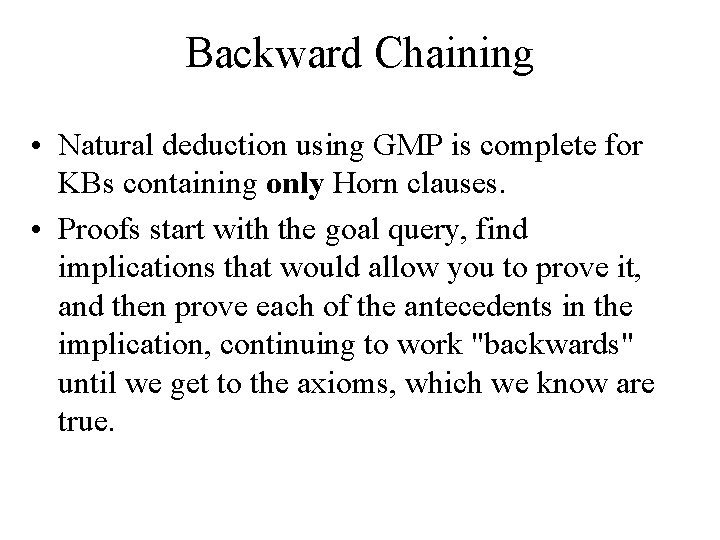Backward Chaining • Natural deduction using GMP is complete for KBs containing only Horn clauses. • Proofs start with the goal query, find implications that would allow you to prove it, and then prove each of the antecedents in the implication, continuing to work "backwards" until we get to the axioms, which we know are true.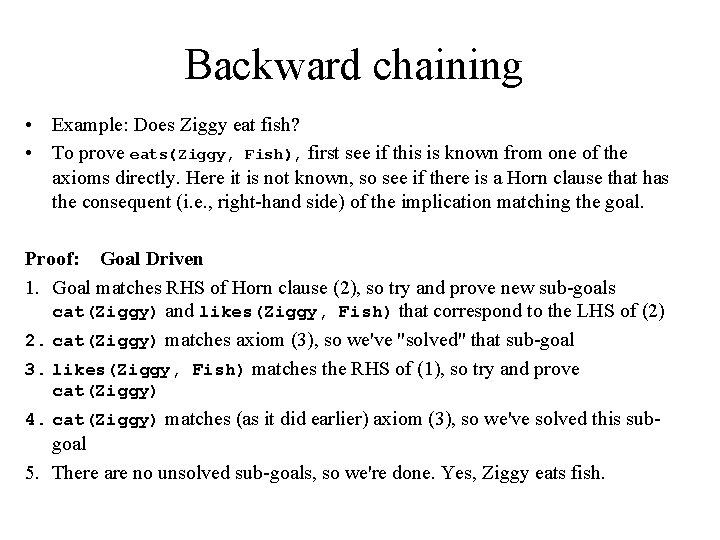Backward chaining • Example: Does Ziggy eat fish? • To prove eats(Ziggy, Fish), first see if this is known from one of the axioms directly. Here it is not known, so see if there is a Horn clause that has the consequent (i. e. , right-hand side) of the implication matching the goal. Proof: Goal Driven 1. Goal matches RHS of Horn clause (2), so try and prove new sub-goals cat(Ziggy) and likes(Ziggy, Fish) that correspond to the LHS of (2) 2. cat(Ziggy) matches axiom (3), so we've "solved" that sub-goal 3. likes(Ziggy, Fish) matches the RHS of (1), so try and prove cat(Ziggy) 4. cat(Ziggy) matches (as it did earlier) axiom (3), so we've solved this sub- goal 5. There are no unsolved sub-goals, so we're done. Yes, Ziggy eats fish.